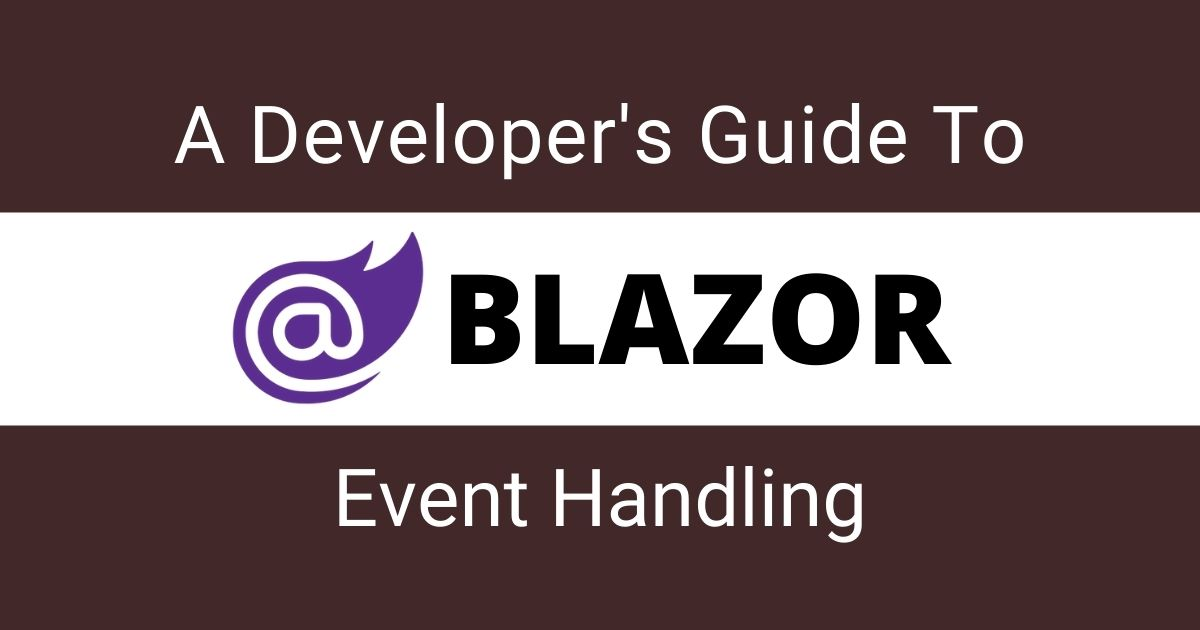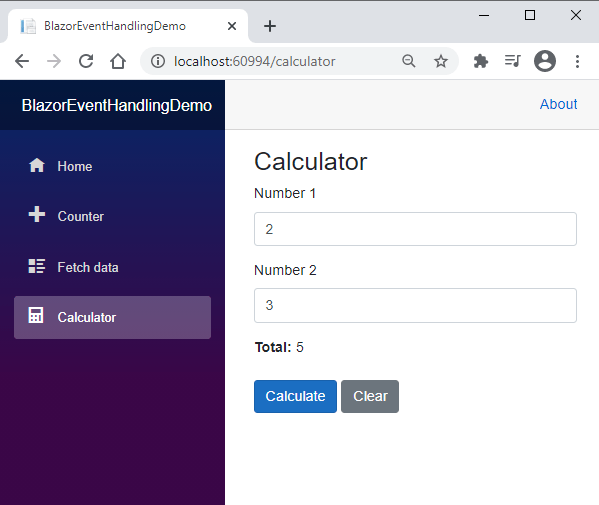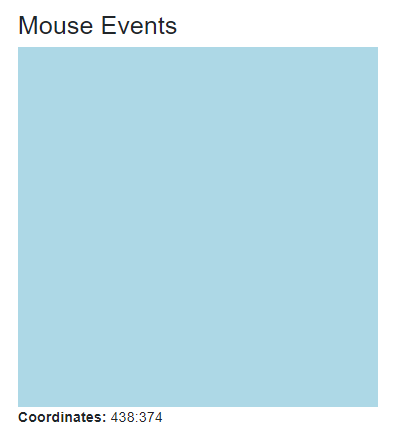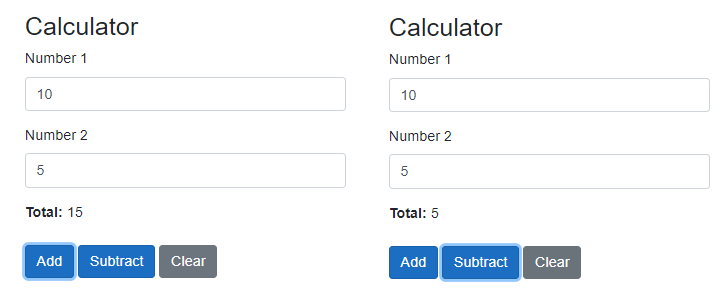## Blazor 事件处理入门

Blazor 中处理事件的基本语法如下所示：

``````@on[DOM EVENT]="[DELEGATE]"
``````

• [DOM EVENT] 是 DOM 事件的占位符，例如 click、mouseup 等。
• [DELEGATE] 是 C# 委托事件处理程序的占位符。

``````<button @onclick="Update" />
``````

``````@page "/calculator"

<h3>Calculator</h3>

<div class="form-group">
<label for="number1">Number 1</label>
<input type="number" class="form-control" id="number1" @bind="number1">
</div>
<div class="form-group">
<label for="number2">Number 2</label>
<input type="number" class="form-control" id="number2" @bind="number2">
</div>
<div class="form-group">
<label><b>Total: </b>@total</label>
</div>

<button class="btn btn-primary" @onclick="Calculate">Calculate</button>
<button class="btn btn-secondary" @onclick="Clear">Clear</button>

@code {
private int number1 = 0;
private int number2 = 0;
private int total = 0;

private void Calculate()
{
total = number1 + number2;
}

private void Clear()
{
number1 = 0;
number2 = 0;
total = 0;
}
}
``````

``````<button class="btn btn-primary" @onclick="Calculate">Calculate</button>
<button class="btn btn-secondary" @onclick="Clear">Clear</button>
````````````private async Task Clear()
{

number1 = 0;
number2 = 0;
total = 0;
}
``````

## 理解 Blazor 事件参数

``````@page "/mouseevents"

<h3>Mouse Events</h3>

<div style="width: 400px; height: 400px; background: lightblue" @onmousemove="Move"></div>
<label><b>Coordinates: </b>@coordinates</label>

@code {
private string coordinates = "";

private void Move(MouseEventArgs e)
{
coordinates = \$"{e.ScreenX}:{e.ScreenY}";
}
}
``````Blazor 支持大量的 EventArgs 对象，但最常用的 EventArgs 如下表所示：

## 在 Blazor 事件中使用 Lambda 表达式

Blazor 还支持将 Lambda 表达式作为委托事件处理程序。您应当只在简单的用例中使用这些表达式，如果有很多的代码要执行，应避免使用 Lambda 表达式。让我们修改一下前面的 Calculator 示例，这次使用 Lambda 表达式替代上面的 CalculateClear 方法。

``````@page "/calculator"
<h3>Calculator</h3>
<div class="form-group">
<label for="number1">Number 1</label>
<input type="number" class="form-control" id="number1" @bind="number1">
</div>
<div class="form-group">
<label for="number2">Number 2</label>
<input type="number" class="form-control" id="number2" @bind="number2">
</div>
<div class="form-group">
<label><b>Total: </b>@total</label>
</div>

<button class="btn btn-primary" @onclick="@(e => total = number1 + number2)">Calculate</button>
<button class="btn btn-secondary" @onclick="@(e => total = number1 = number2 = 0)">Clear</button>

@code {
private int number1 = 0;
private int number2 = 0;
private int total = 0;
}
``````

## 向事件处理程序传递附加参数

``````<div class="form-group">
<label for="number1">Number 1</label>
<input type="number" class="form-control" id="number1" @bind="number1">
</div>

<div class="form-group">
<label for="number2">Number 2</label>
<input type="number" class="form-control" id="number2" @bind="number2">
</div>

<div class="form-group">
<label><b>Total: </b>@total</label>
</div>

<button class="btn btn-primary" @onclick="@(e => Calculate(e, 1))">Add</button>
<button class="btn btn-primary" @onclick="@(e => Calculate(e, 2))">Subtract</button>

<button class="btn btn-secondary" @onclick="Clear">Clear</button>

@code {
private int number1 = 0;
private int number2 = 0;
private int total = 0;

private void Calculate(MouseEventArgs e, int buttonType)
{
switch (buttonType)
{
case 1:
total = number1 + number2;
break;
case 2:
total = number1 - number2;
break;
}
}

private void Clear()
{
number1 = 0;
number2 = 0;
total = 0;
}
}
``````

``````<button class="btn btn-primary" @onclick="@(e => Calculate(e, 1))">Add</button>
<button class="btn btn-primary" @onclick="@(e => Calculate(e, 2))">Subtract</button>
``````

``````private void Calculate(MouseEventArgs e, int buttonType)
{
switch (buttonType)
{
case 1:
total = number1 + number2;
break
case 2:
total = number1 - number2;
break;
}
}
``````